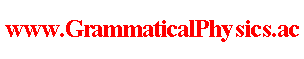since 2006 Help　Sitemap < Forum > < Problems > < Quantum History Theory > < Exact Solution of Uda Equation (2b) > Encouraged by the success of Exact Solution of Uda Equation (2) @ Quantum History Theory @ Products, I began to hope that any classical motion appears asymptotically generally as a delta function factor in a solution. In this page, I try to write such a solution for harmonic oscillator problem. x(t) = A sin(ωt - B), x(a) = A sin(ωa - B), x(b) = A sin(ωb - B). ωa - B = sin-1[x(a)/A], ωb - B = sin-1[x(b)/A], ω(b - a) = sin-1[x(b)/A] - sin-1[x(a)/A], sin[ω(b - a)] = [x(b)/A]cos{sin-1[x(a)/A]} - cos{sin-1[x(b)/A]}[x(a)/A] 　= ±[x(b)/A]√{1 - [x(a)/A]2} ± [x(a)/A]√{1 - [x(b)/A]2} 　= ±[ [x(b)/A]√{1 - [x(a)/A]2} + [x(a)/A]√{1 - [x(b)/A]2} ] 　　　or ±[ [x(b)/A]√{1 - [x(a)/A]2} - [x(a)/A]√{1 - [x(b)/A]2} ] A2sin[ω(b - a)] = ±{x(b)√[A2 - x(a)2] + x(a)√[A2 - x(b)2]} 　　　or ±{x(b)√[A2 - x(a)2] - x(a)√[A2 - x(b)2]}. How about putting as follows? Φ[χ] = δ( lima→-∞limb→+∞{χ(b)√[A2 - χ(a)2] + χ(a)√[A2 - χ(b)2] - A2sin[ω(b - a)]} )F[χ]. For the present, I decided to stop going on this way more because of too less hope.(2019/10/14) Author Yuichi Uda, Write start at 2019/10/13/15:10JST, Last edit at 2019/10/14/14:45JST